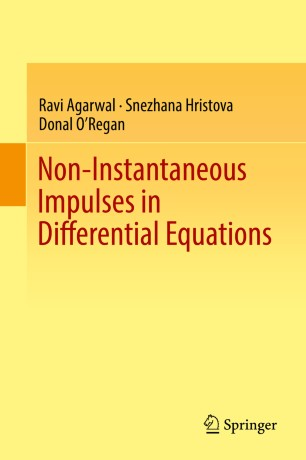# Non-Instantaneous Impulses in Differential Equations

• Ravi Agarwal
• Snezhana Hristova
• Donal O'ReganBook

1. Front Matter
Pages i-xi
2. Ravi Agarwal, Snezhana Hristova, Donal O’Regan
Pages 1-72
3. Ravi Agarwal, Snezhana Hristova, Donal O’Regan
Pages 73-192
4. Ravi Agarwal, Snezhana Hristova, Donal O’Regan
Pages 193-244
5. Back Matter
Pages 245-251

### Introduction

This monograph is the first published book devoted to the theory of differential equations with non-instantaneous impulses. It aims to equip the reader with mathematical models and theory behind real life processes in physics, biology, population dynamics, ecology and pharmacokinetics. The authors examine a wide scope of differential equations with non-instantaneous impulses through three comprehensive chapters, providing an all-rounded and unique presentation on the topic, including:

- Ordinary differential equations with non-instantaneous impulses (scalar and n-dimensional case)
- Fractional differential equa
tions with non-instantaneous impulses (with Caputo fractional derivatives of order q ϵ (0, 1))
- Ordinary differential equations with non-instantaneous impulses occurring at random moments (with exponential, Erlang, or Gamma distribution)

Each chapter focuses on theory, proofs and examples, and contains numerous graphs to enrich the reader’s understanding. Additionally, a carefully selected bibliography is included. Graduate students at various levels as well as researchers in differential equations and related fields will find this a valuable resource of both introductory and advanced material.

### Keywords

partial differential equations ordinary differential equations non-instantaneous impulses fractional derivatives fractional differential equations Lyapunov functions differential equations Caputo fractional derivatives non-instantaneous impulses in differential equations impulse systems

#### Authors and affiliations

• Ravi Agarwal
• 1
• Snezhana Hristova
• 2
• Donal O'Regan
• 3
1. 1.Department of MathematicsTexas A&M University—KingsvilleKingsvilleUSA
2. 2.Department of Applied MathematicsPlovdiv UniversityPlovdivBulgaria
3. 3.School of Mathematics, Statistics and Applied MathematicsNational University of IrelandGalwayIreland